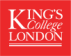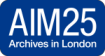Cambridge University Libraries are providing a blend of online and controlled in-person services. Please see our website for more details.

# Treatise of fluxions, 1758

File
Reference Code: GBR/0180/RGO 4/242

## Scope and Contents

The original manuscript of Israel Lyons' 'Treatise of Fluxions', divided into twelve sections.

1. The direct method of fluxions: i. Definitions; ii. Fluxions of the sum and differences of quantities; iii. Fluxions of rectangles and powers; iv. To find the relation of the fluxion from the relation of the fluents; v. Of 2nd, 3rd and 4th fluxions.

2. The inverse method of fluxions: i. Of the fluents of simple quantities; ii. Newton's binomial theorem demonstrated; iii. Demoivre's multinomial theorem and quantities; iv. Of the fluents of compound and surd quantity; v. Theorems for finding fluents; vi. Finding fluents by successive fluxions and fluents; vii. Of the resolution of adfected literal equations; viii. Of the resolution of fluxional equations; ix. Reversion of series.

3. LogaritHMS and measures of ratios: i. Composition of ratios; ii. To find the measure of any ratio; iii. Series for finding logaritHMS; iv. Of exponentials; v. Finding of fluents by the measures of ratios.

4. Of curves and drawing of tangents: i. Definitions; ii. Of lines of the first and second order; iii. Of the conchoid of Nicomedes; iv. Of the cissoid of Diocles, paraboloids and hyperboloides; v. To draw tangents to any curve; vi. To draw tangents to the conic sections; vii. To draw tangents to the conchoid; viii. To draw tangents to the cissoid; ix. To draw tangents to paraboloids and hyperboloides; x. Of mechanical curves; xi. Of the logarithmic curve; xii. Of cycloids; xiii. Of spirals; xiv. Of the quadratrix.

5. Of the greatest and least ordinates: i. Of Tayler's theorem; ii. To find when an ordinate becomes a maximum or minimum of the first kind; iii. Of the second kind; iv. Examples; v. Of the curve of swiftest descent; vi. To draw a perpendicular to a curve.

6. Of the curvature of curves: i. Curvature of different circle; ii. To find the radius of curvature in any curve; iii. Of the evoluta; iv. Variations of curvatures; v. To find the curvature of a conic section; vi. To find the curvature of a conchoid; vii. To find the curvature of a cissoid; viii. To find the curvature of a paraboloid; ix. To find the curvature of a logarithmic curve; x. To find the curvature of a cycloid; xi. To find the curvature of a quadratrix; xii. To find the quadrature in the curve of swiftest descent; xiii. To find the curvature of spirals; xiv. To find the curvature of the epicycloid; xv. Of the curvature in the figure mentioned by Sir Isaac Newton, 'Princip Lib 3 prop 28'; xvi. To investigate the curve in which the radius of curvature is inversely as any power of the ordinates.

7. Of the points of contrary flexion: i. Definition; ii. Examples in the conchoid, paraboloid and cycloid; iii. To find the points of contrary flexure in a spiral; iv. Of cuspids; v. Examples in the cissoid and paraboloid.

8. Of the quadrature of curves: i. Comparison of fluents; ii. Of the fluxions of curvilinear areas; iii. To find the area of the parabola, paraboloid, hyperbola and circle; iv. Of fluents that may be compared with the circle; v. To find the area of an ellipsis and hyperbola; vi. Of fluents that may be compared with the hyperbola, cissoid, conchoid, logarithmic curve, cycloid and quadratrix; vii. To find the area when the equation is an adfected equation, and the area of a spiral; viii. Compute the area of the differences of the fluxions of the ordinates; ix. To compute the area by equidistant ordinates.

9. The rectification of curve lines: i. To find the length of a parabola, paraboloid, circle by infinite series, and the circle by logaritHMS; ii. The demonstration of Mr Cotes' theorem; iii. To find the length of an ellipse, hyperbola, cissoid, conchoid, logarithmic curve, cycloid, quadratrix and spirals.

10. Of the contents of solids: i. Fluxions of solids; ii. The content of the solid generated by a paraboloid, ellipse and hyperbola, cissoid, conchoid, logarithmic curve and quadratrix.

11. Of the quadrature of curvilinear surfaces: i. The surface of a cone; ii. The fluxions of curved surfaces; iii. Area of the surface generated by a paraboloid; iv. Surfaces of a sphere, hyperbolic conoid, spheroid, cissoid and logarithmic curve; v. When a surface is finite and when infinite.

12. Of the centre of gravity: i. To find the centre of gravity of a paraboloid, circle and ellipse, hyperbola, circular arc, solid described by a paraboloid, segment of a sphere or spheroid and hyperbolic conoid; ii. Centre of oscillation; iii. To find the centre of oscillation of a right line, circle, sphere and paraboloid; iv. Of the casenaria; v. Solutions to some logometrical problems.

• 1758

## Conditions Governing Access

Unless restrictions apply, the collection is open for consultation by researchers using the Manuscripts Reading Room at Cambridge University Library. For further details on conditions governing access please contact mss@lib.cam.ac.uk. Information about opening hours and obtaining a Cambridge University Library reader's ticket is available from the Library's website (www.lib.cam.ac.uk).

## Extent

1 volume(s) (1 volume)

## Language of Materials

English

A2/421O

### Finding aid date

2005-11-07 13:43:12+00:00

Includes index.

## Repository Details

### Repository Details

Part of the Cambridge University Library Repository

Contact:
Cambridge University Library